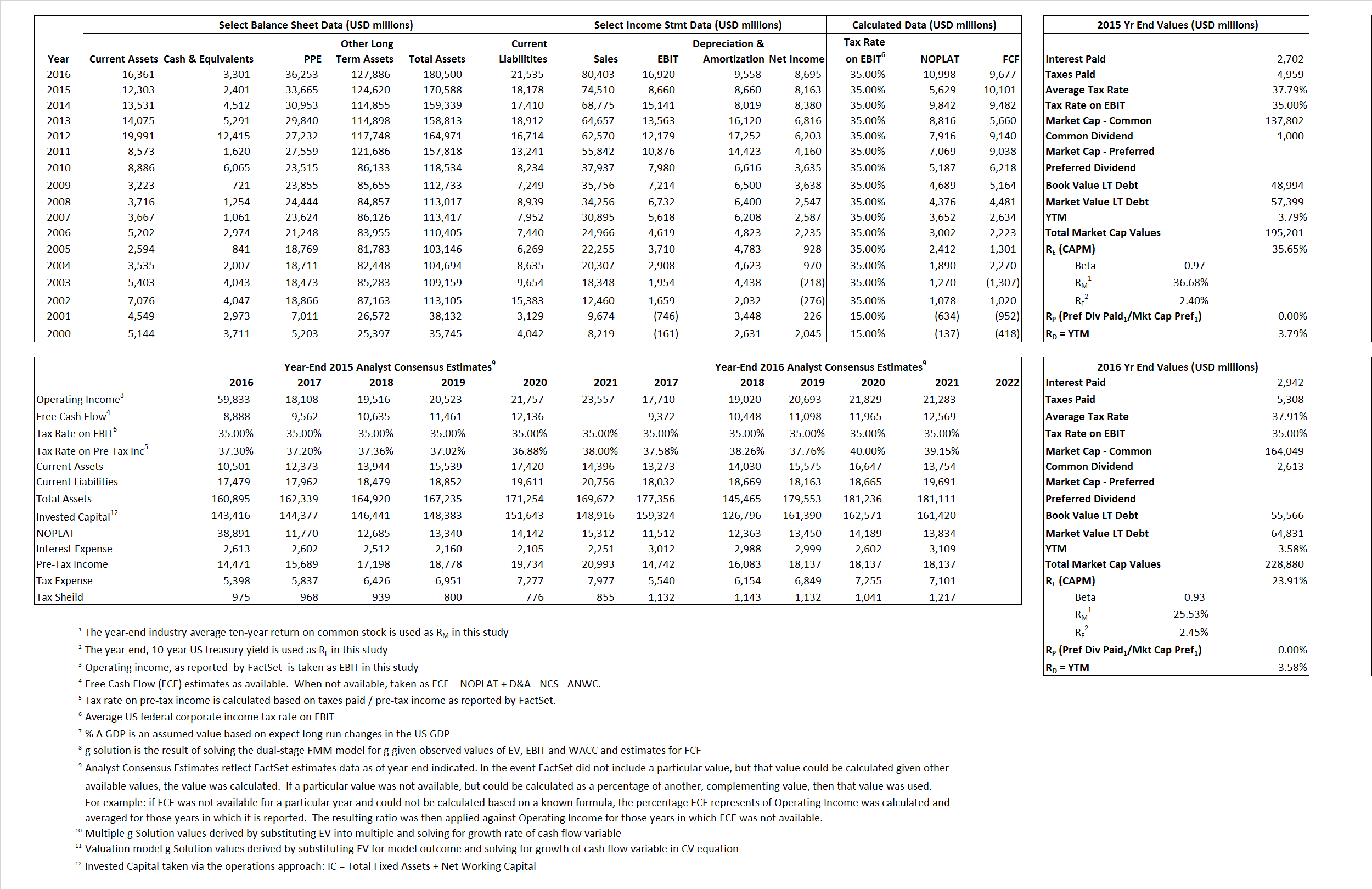# Comcast Corporation Class A

## Analyst Listing

The following analysts provide coverage for the subject firm as of May 2016:

 Broker Analyst Analyst Email Pacific Crest Securities-KBCM Andy Hargreaves ahargreaves@pacific-crest.com Nomura Research Anthony DiClemente anthony.diclemente@nomura.com Deutsche Bank Research Bryan Kraft bryan.kraft@db.com Raymond James Frank G. Louthan IV frank.louthan@raymondjames.com Atlantic Equities Hamiton Faber h.faber@atlantic-equities.com Buckingham Research James Ratcliffe jratcliffe@buckresearch.com Scotiabank GBM Jeffrey Fan jeff.fan@scotiabank.com Pivotal Research Group Jeffrey Wlodarczak jeff@pvtl.com RBC Capital Markets Jonathan Atkin jonathan.atkin@rbccm.com Wells Fargo Securities Marci Ryvicker marci.ryvicker@wellsfargo.com Wunderlich Securities Matthew Harrigan mharrigan@wundernet.com Guggenheim Securities Michael C. Morris michael.morris@guggenheimpartners.com Jefferies Mike McCormack mmccormack@jefferies.com Credit Suisse Omar Sheikh omar.sheikh@credit-suisse.com Oppenheimer Timothy Horan tim.horan@opco.com Evercore ISI Vijay Jayant vijay.jayant@evercoreisi.com

## Primary Input Data## Derived Input Data

### Equational Form

Net Operating Profit Less Adjusted Taxes NOPLAT  5,629 10,998$NOPLAT\, =\, EBIT\, x\, (1 \,-\, Avg \,\,Tax\,\, Rate\,\, on\,\, EBIT)$
Free Cash Flow FCF 10,101 9,677$FCF\,=NOPLAT\,+\,Non-Cash\,Expenses-\Delta NWC\,-\,NCS$
Tax Shield TS 1,021 1,115$TS\,=\,Interest\,\,Paid\,\,x\,\, Avg \,\,Tax\,\,Rate\,\, on\,\, Pre-Tax\,\, Income$
Invested Capital IC 152,410 158,965$IC\,=\,Fixed\,\,Operating\,\,Assets\,\,+\,\,Net\,\, Working\,\, Capital$
Return on Invested Capital ROIC 3.69% 6.92%$ROIC\,=\,\frac { NOPLAT }{ IC }$
Net Investment NetInv 19,141 16,113$NetInv\,=\,{ {IC}_{1}}-{{IC}_{0}}+Depreciation$
Investment Rate IR  340.04% 146.51%$IR\,=\,\frac {NetInv}{NOPLAT}$
Weighted Average Cost of Capital
WACCMarket 25.86% 17.77%$WACC\,=\,\frac { E }{ V } { R }_{ E }\,+\,\frac { P }{ V } { R }_{ P }\,+\,\frac { D }{ V } { R }_{ D }\left( 1- Avg\,\, Tax\,\,Rate\,\,on\,\,Pre-Tax\,\,Income \right)$
WACCBook  7.13%  6.74%
Enterprise value
EVMarket  192,800  225,579$EV\,=\,Market\,\,Cap\,\,Equity\,+\,\,Long\,\,Term\,\,Debt\,-\,Cash$
EVBook  184,395  216,314
Long-Run Growth
g = IR x ROIC
12.56%  10.14% Long-run growth rates of the income variable are used in the Continuing Value portion of the valuation models.
g = %$\Delta$ GDP    2.50%    2.50%
Margin from Operations M 11.62%  21.04%$M\,\,=\,\,\frac{EBIT}{SALES}$
Depreciation/Amortization Rate D 50.00%  36.10%$D\,\,=\,\,\frac{D+A}{EBITDA}$

## Valuation Multiple Outcomes

The outcomes presented in this study are the result of original input data, derived data, and synthesized inputs.

### model g solution

12/31/2015 12/31/2016 12/31/2015 12/31/2016 12/31/2015 12/31/2016

EV/SALES$\frac {EV}{Sales} \,= \,\frac{ROIC\, -\, g}{ROIC\,(WACC\,-\,g)}\,(1\,-\,T)\,(M)$

2.59  2.81  109.51%  43.67%  34.95%  22.67%

EV/EBITDA$\frac {EV}{EBITDA} \,= \,\frac{ROIC\, -\, g}{ROIC\,(WACC\,-\,g)}\,(1\,-\,T)\,(1\,-\,D)$

11.13 8.52 109.51% 43.67% 34.95% 22.67%

EV/NOPLAT$\frac {EV}{NOPLAT} \,= \,\frac{ROIC\, -\, g}{ROIC\,(WACC\,-\,g)}$

34.25 20.51 109.51% 43.67% 34.95% 22.67%

EV/FCFOPS$\frac {EV}{FCF_{OPS}} \,= \,\frac{ROIC\, -\, g}{ROIC\,(WACC\,-\,g)}\,(1\,-\,T)$

19.09 23.31 109.51% 43.67% 34.95% 22.67%

EV/EBIT$\frac {EV}{EBIT} \,= \,\frac{ROIC\, -\, g}{ROIC\,(WACC\,-\,g)}\,(1\,-\,T)$

22.26 13.33 109.51% 43.67% 34.95% 22.67%

EV/IC$\frac {EV}{IC} \,= \,\frac{ROIC\, -\, g}{WACC\,-\,g}$

1.27 1.42 109.51% 43.67% 34.95% 22.67%